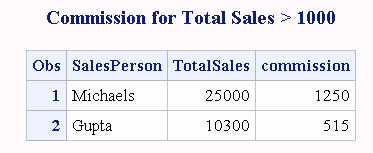# IFN Function

Returns a numeric value based on whether an expression is true, false, or missing.

 Category: Numeric Restriction: I18N Level 2 functions are designed for use with SBCS, DBCS, and MBCS (UTF8).

## Syntax

### Required Arguments

#### logical-expression

specifies a numeric constant, variable, or expression.

#### value-returned-when-true

specifies a numeric constant, variable, or expression that is returned when the value of logical-expression is true.

#### value-returned-when-false

specifies a numeric constant, variable, or expression that is returned when the value of logical-expression is false.

### Optional Argument

#### value-returned-when-missing

specifies a numeric constant, variable or expression that is returned when the value of logical-expression is missing.

## Details

The IFN function uses conditional logic that enables you to select among several values based on the value of a logical expression.
IFN evaluates the first argument, then logical-expression. If logical-expression is true (that is, not zero and not missing), then IFN returns the value in the second argument. If logical-expression is a missing value, and you have a fourth argument, then IFN returns the value in the fourth argument. Otherwise, if logical-expression is false, IFN returns the value in the third argument.
The IFN function, an IF/THEN/ELSE construct, or a WHERE statement can produce the same results. (See examples.) However, the IFN function is useful in DATA step expressions when it is not convenient or possible to use an IF/THEN/ELSE construct or a WHERE statement.

## Comparisons

The IFN function is similar to the IFC function, except that IFN returns a numeric value whereas IFC returns a character value.

## Examples

### Example 1: Calculating Commission Using the IFN Function

In the following example, IFN evaluates the expression `TotalSales > 10000`. If total sales exceeds \$10,000, then the sales commission is 5% of the total sales. If total sales is less than \$10,000, then the sales commission is 2% of the total sales.
```data _null_;
input TotalSales;
commission=ifn(TotalSales > 10000, TotalSales*.05, TotalSales*.02);
put commission=;
datalines;
25000
10000
500
10300
;
run;```
SAS writes the following output to the log:
```commission=1250
commission=200
commission=10
commission=515```

### Example 2: Calculating Commission Using an IF/THEN/ELSE Construct

In the following example, an IF/THEN/ELSE construct evaluates the expression ```TotalSales > 10000```. If total sales exceeds \$10,000, then the sales commission is 5% of the total sales. If total sales is less than \$10,000, then the sales commission is 2% of the total sales.
```data _null_;
input TotalSales;
if TotalSales > 10000 then commission = .05 * TotalSales;
else commission = .02 * TotalSales;
put commission=;
datalines;
25000
10000
500
10300
;
run;```
SAS writes the following output to the log:
```commission=1250
commission=200
commission=10
commission=515```

### Example 3: Calculating Commission Using a WHERE Statement

In the following example, a WHERE statement evaluates the expression ```TotalSales > 10000```. If total sales exceeds \$10,000, then the sales commission is 5% of the total sales. If total sales is less than \$10,000, then the sales commission is 2% of the total sales. The output shows only those salespeople whose total sales exceed \$10,000.
```data sales;
input SalesPerson \$ TotalSales;
datalines;
Michaels 25000
Janowski 10000
Chen 500
Gupta 10300
;
data commission;
set sales;
where TotalSales > 10000;
commission = TotalSales * .05;
run;
proc print data=commission;
title 'Commission for Total Sales > 1000';
run;```
Output from a WHERE Statement